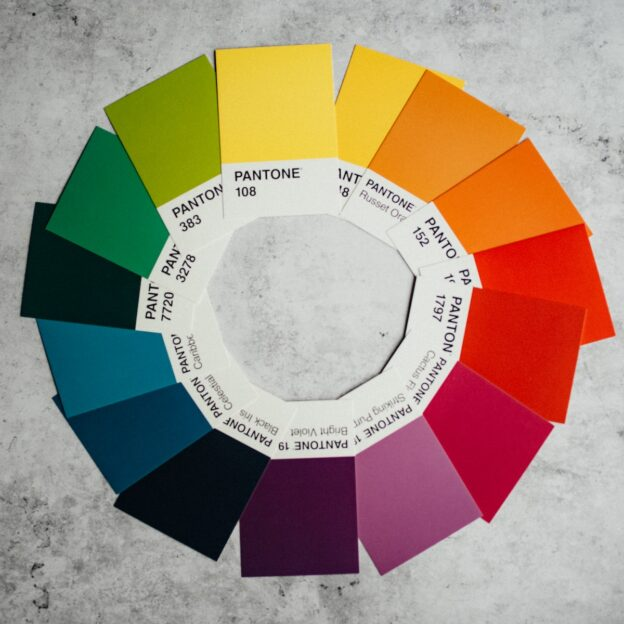HSL 是将 RGB 三维坐标转换为色相、饱和度、亮度（英语：Hue, Saturation, Lightness）组成的新维度坐标。

## RGB to HSL 算法

https://zh.wikipedia.org/wiki/HSL%E5%92%8CHSV%E8%89%B2%E5%BD%A9%E7%A9%BA%E9%97%B4h的值通常规范化到位于0到360°之间。而h = 0用于max = min的（定义为灰色）时候而不是留下h未定义。

## RGB to HSL Sample Code

function rgbToHsl(r, g, b) {
r /= 255, g /= 255, b /= 255;

var max = Math.max(r, g, b),
min = Math.min(r, g, b);
var h, s, l = (max + min) / 2;

if (max == min) {
h = s = 0; // achromatic
} else {
var d = max - min;
s = l > 0.5 ? d / (2 - max - min) : d / (max + min);

switch (max) {
case r:
h = (g - b) / d + (g < b ? 6 : 0);
break;
case g:
h = (b - r) / d + 2;
break;
case b:
h = (r - g) / d + 4;
break;
}

h /= 6;
}

return [h, s, l];
}

## 延展阅读

https://www.december.com/html/spec/colorterms.html